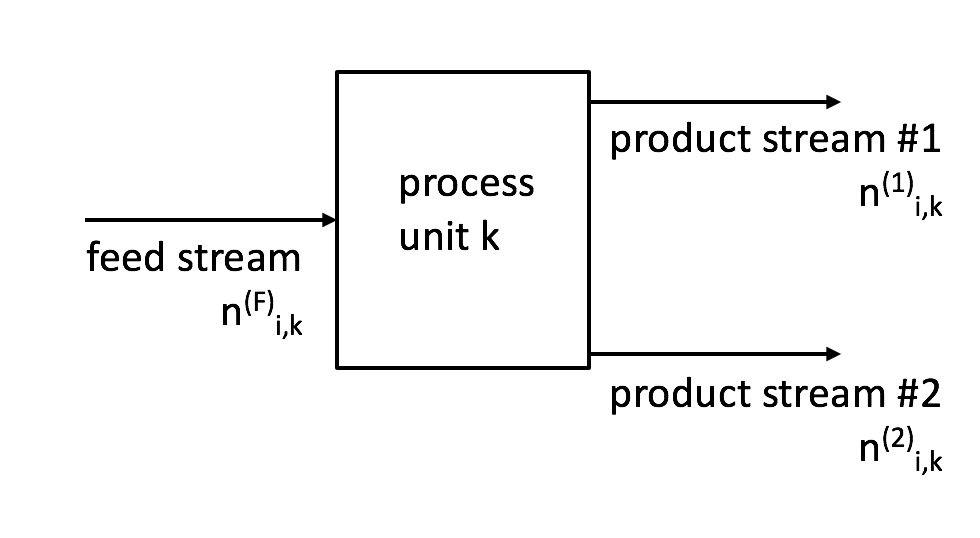# 1.1: Performance Metrics for Separation Processes

$$\newcommand{\vecs}{\overset { \rightharpoonup} {\mathbf{#1}} }$$ $$\newcommand{\vecd}{\overset{-\!-\!\rightharpoonup}{\vphantom{a}\smash {#1}}}$$$$\newcommand{\id}{\mathrm{id}}$$ $$\newcommand{\Span}{\mathrm{span}}$$ $$\newcommand{\kernel}{\mathrm{null}\,}$$ $$\newcommand{\range}{\mathrm{range}\,}$$ $$\newcommand{\RealPart}{\mathrm{Re}}$$ $$\newcommand{\ImaginaryPart}{\mathrm{Im}}$$ $$\newcommand{\Argument}{\mathrm{Arg}}$$ $$\newcommand{\norm}{\| #1 \|}$$ $$\newcommand{\inner}{\langle #1, #2 \rangle}$$ $$\newcommand{\Span}{\mathrm{span}}$$ $$\newcommand{\id}{\mathrm{id}}$$ $$\newcommand{\Span}{\mathrm{span}}$$ $$\newcommand{\kernel}{\mathrm{null}\,}$$ $$\newcommand{\range}{\mathrm{range}\,}$$ $$\newcommand{\RealPart}{\mathrm{Re}}$$ $$\newcommand{\ImaginaryPart}{\mathrm{Im}}$$ $$\newcommand{\Argument}{\mathrm{Arg}}$$ $$\newcommand{\norm}{\| #1 \|}$$ $$\newcommand{\inner}{\langle #1, #2 \rangle}$$ $$\newcommand{\Span}{\mathrm{span}}$$

$$n_{i,k}^{(F)}$$ = mass or molar flow rate of species $$i$$ entering separator $$k$$ in feed stream (mass time-1 or mol time-1)

$$n_{i,k}^{(1)}$$ = mass or molar flow rate of species $$i$$ leaving separator $$k$$ in first product stream (mass time-1 or mol time-1)

$$n_{i,k}^{(2)}$$ = mass or molar flow rate of species $$i$$ leaving separator $$k$$ in second product stream (mass time-1 or mol time-1)

$${\rm SF}_{i,k}$$ = split fraction for species $$i$$ in separator $$k$$ (dimensionless)

$${\rm SR}_{i,k}$$ = split ratio for species $$i$$ in separator $$k$$ (dimensionless)$\tag{1.1} {\rm SF}_{i,k} = n_{i,k}^{(1)} / n_{i,k}^{(F)}$

$\tag{1.2} {\rm SR}_{i,k} = n_{i,k}^{(1)}/n_{i,k}^{(2)} = {\rm SF}_{i,k} / (1-{\rm SF}_{i,k})$

## Example

For the process below, compute the split fraction and the split ratio for $$i C_5 H_{12}$$. Designate the overhead stream as product stream 1.This page titled 1.1: Performance Metrics for Separation Processes is shared under a CC BY-NC 4.0 license and was authored, remixed, and/or curated by Monica Lamm and Laura Jarboe (Iowa State University Digital Press) via source content that was edited to the style and standards of the LibreTexts platform; a detailed edit history is available upon request.